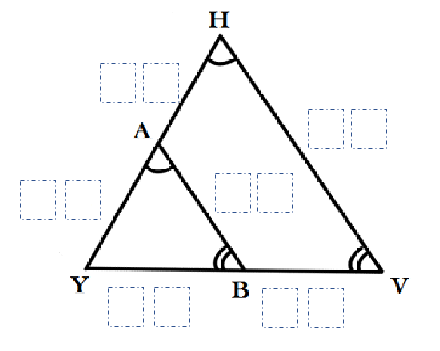# Similar Triangles

Directions: Using the digits 0 to 9 at most one time each and as many leading zeros as you like, place a digit in each box to create two similar triangles.### Hint

Can you use the scale factor to help you in any way?
What is true about corresponding sides of similar triangles?

YA – 5
AH – 10
YB – 3
BV – 6
BA – 9
VH – 27

Source: Drew Ross

## Trigonometric Ratios

Directions: Using the digits 0 to 9 at most one time each, place a digit …

1.I believe the correct answer to VH should be 18 given that the ratios between the 2 corresponding sides is 1 : 2

So, the corresponding sides are 3 : 6 , 5 : 10 and therefore the 3 pair of corresponding sides should be 9 : 18?

Please confirm or show where I made my mistake in my calculation or assumption?

2.I believe the correct answer to VH should be 18 given that the ratios between the 2 corresponding sides is 1 : 2

So, the corresponding sides are 3 : 6 , 5 : 10 and therefore the 3 pair of corresponding sides should be 9 : 18?

Please confirm or show where I made my mistake in my calculation or assumption?

•AH & BV are not side lengths. They are only part of those sides in the bigger triangle.

3.If you look at triangle AYB, the proposed sides of 5, 3 and 9 do not form a triangle since 5 + 3 < 9.

One of my students found:
YA – 5
AH – 10
YB – 4
BV – 8
BA – 2
VH – 6

4.I think this one is improved by removing the label on AH. It’s already restricted by both the scale factor and the triangle inequality (as noted by Lori). If you remove AH, then you can get non-integer scale factors such as AB:HV = 30:48.